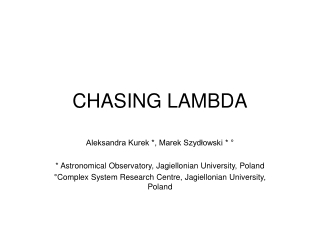DownloadDownload PresentationCHASING LAMBDA

# CHASING LAMBDA

Télécharger la présentation## CHASING LAMBDA

- - - - - - - - - - - - - - - - - - - - - - - - - - - E N D - - - - - - - - - - - - - - - - - - - - - - - - - - -
##### Presentation Transcript

1. CHASING LAMBDA Aleksandra Kurek *, Marek Szydłowski * ° * Astronomical Observatory, Jagiellonian University, Poland °Complex System Research Centre, Jagiellonian University, Poland

2. MODELS WITH DARK ENERGY (Weinberg 1989) (Caldwell 2002) (Chevallier & Polarski 2001) (Peebles & Ratra 1988) ≡ (Rahvar & Movahed 2007) mean of the coefficient of the EQS in the log scale factor

3. MODELS WITH MODIFIED THEORY OF GRAVITY (Dvali et al. 2000) (Singh & Vandersloot 2005) (Szydlowski et al. 2006) ; (Freese & Lewis 2002) (Shtanov 2000)

4. BAYESIAN FRAMEWORK OF MODEL SELECTION

5. BAYESIAN FRAMEWORK OF MODEL SELECTION vector of model parameters likelihood of the model prior probability for model parameters approximation to -2 ln E number of data points maximum likelihood number of model parameters assumptions • iid • 3. prior for model parameters ≠ 0 in the • maximum likelihood • 4.sample size large with respect to the number • of model parameters 2. 1.prior for model parameters ≠ 0 in the neighborhood of the maximum likelihood 2. prior is bound in the whole parameter space 3.sample size large with respect to the number of model parameters Cavanough & Neath 1999 Schwarz 1978

6. BAYESIAN FRAMEWORK OF MODEL SELECTION POSTERIOR ODDS BAYES FACTOR - B 12 evidence in favor model 1 2 ln B 12 not worth than a bare mention <0,2) <2,6) positive <6,10) strong >10 very strong (Kass & Raftery 1995)

7. APPLICATION TO COSMOLOGICAL MODELS COMPARISON (Riess et al. 2007; Wood-Vasey et al. 2007; Davis et al. 2007) SN R (Spergel et al. 2006; Wang & Mukherjee 2006)

8. APPLICATION TO COSMOLOGICAL MODELS COMPARISON A (Eisenstein et al. 2005) (differential ages of the passively evolving galaxies) H (Simon et al. 2005) L L L L L sn R H A N=192+1+1+9

9. POSTERIOR PROBABILITIES FOR MODELS WITH DARK ENERGY 0.84 0.02 0.06 0.04 0.04

10. POSTERIOR PROBABILITIES FOR MODELS WITH MODIFIED GRAVITY 0.07 0.03 0.13 0.74 0.03

11. POSTERIOR PROBABILITIES FOR ALL MODELS 0.74 0.02 0.05 0.04 0.03 0.01 0.005 0.01 0.09 0.005

12. CONCLUSIONS In the light of data used in analysis: • ΛCDM model is the best one from the set of models with dark energy as well as the best one from the set of all models considered • Cardassian model is the best one from the set of models with modified gravity